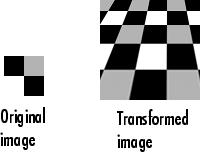N-Dimensional Spatial Transformations

The following functions, when used in combination, provide a vast array of options for defining and working with 2-D, N-D, and mixed-D spatial transformations:

The imtransform, findbounds, and tformarray functions use the tformfwd and tforminv functions internally to encapsulate the forward transformations needed to determine the extent of an output image or array and/or to map the output pixels/array locations back to input locations. You can use tformfwd and tforminv to explore the geometric effects of a transformation by applying them to points and lines and plotting the results. They support a consistent handling of both image and point-wise data.

You can use tformarray to work with arbitrary-dimensional array transformations. The arrays do not need to have the same dimensions. The output can have either a lower or higher number of dimensions than the input. For example, if you are sampling 3-D data on a 2-D slice or manifold, the input array might have a lower dimensionality. The output dimensionality might be higher, for example, if you combine multiple 2-D transformations into a single 2-D to 3-D operation.

You can create a resampling structure using the makeresampler function to obtain special effects or custom processing. For example, you could specify your own separable filtering/interpolation kernel, build a custom resampler around the MATLAB® interp2 or interp3 functions, or even implement an advanced antialiasing technique.

The following example uses imtransform to perform a projective transformation of a checkerboard image, and makeresampler to create a resampling structure with a standard interpolation method.

I = checkerboard(20,1,1);
figure; imshow(I)
T = maketform('projective',[1 1; 41 1; 41 41;   1 41],...
[5 5; 40 5; 35 30; -10 30]);
R = makeresampler('cubic','circular');
K = imtransform(I,T,R,'Size',[100 100],'XYScale',1);
figure, imshow(K)The imtransform function options let you control many aspects of the transformation. For example, note how the transformed image appears to contain multiple copies of the original image. This is accomplished by using the 'Size' option, to make the output image larger than the input image, and then specifying a padding method that extends the input image by repeating the pixels in a circular pattern. The Image Processing Toolbox™ Image Transformation demos provide more examples of using the imtransform function and related functions to perform different types of spatial transformations.